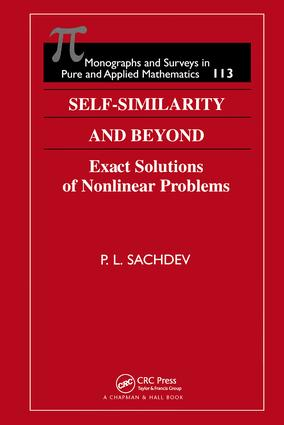# Self-Similarity and Beyond

## Exact Solutions of Nonlinear Problems, 1st Edition

Chapman and Hall/CRC

336 pages | 10 B/W Illus.

Hardback: 9781584882114
pub: 2000-08-24
\$250.00
x
eBook (VitalSource) : 9780429115950
pub: 2019-06-13
from \$28.98

FREE Standard Shipping!

### Description

Nonlinearity plays a major role in the understanding of most physical, chemical, biological, and engineering sciences.

Nonlinear problems fascinate scientists and engineers, but often elude exact treatment. However elusive they may be, the solutions do exist-if only one perseveres in seeking them out.

Self-Similarity and Beyond presents a myriad of approaches to finding exact solutions for a diversity of nonlinear problems. These include group-theoretic methods, the direct method of Clarkson and Kruskal, traveling waves, hodograph methods, balancing arguments, embedding special solutions into a more general class, and the infinite series approach.

The author's approach is entirely constructive. Numerical solutions either motivate the analysis or confirm it, therefore they are treated alongside the analysis whenever possible. Many examples drawn from real physical situations-primarily fluid mechanics and nonlinear diffusion-illustrate and emphasize the central points presented.

Accessible to a broad base of readers, Self-Similarity and Beyond illuminates a variety of productive methods for meeting the challenges of nonlinearity. Researchers and graduate students in nonlinearity, partial differential equations, and fluid mechanics, along with mathematical physicists and numerical analysts, will re-discover the importance of exact solutions and find valuable additions to their mathematical toolkits.

### Reviews

"The main theme of this book is exact solutions to nonlinear partial differential equations and systematic methods for finding them. All techniques are demonstrated with plenty of worked-out examples, which are predominantly drawn from fluid mechanics, reaction-diffusion systems, and nonlinear diffusion…Throughout the book, notation is kept elementary, and the sections are largely independent of each other. This work will serve as a valuable reference for anybody working in applied mathematics and respective areas of application, as well as a source of nontrivial, yet elementary, examples of solutions to nonlinear partial differential equations for teaching purposes."

- Mathematical Reviews, Issue 2002

"The methods and their limitations are clearly explained, and are complemented by a number of solved examples focusing on equations in fluid mechanics and nonlinear diffusion."

-European Mathematical Society Newsletter, No. 42, December 2001

INTRODUCTION

FIRST ORDER PARTIAL DIFFERENTIAL EQUATIONS

Linear Partial Differential Equations of First Order

Quasilinear Partial Differential Equation of First Order

Reduction of ut = unux+H(x,t,u)=0 to form Ut + UnnUx=0

Initial Value problem for ut+ g(u)Ux+lh(u)=0

Initial Value problem for ut+ ua+ux+ lub=0

EXACT SIMILARITY SOLUTIONS OF NONLINEAR PDES

Reduction of PDEs by Infinitesimal Transformation

System of Partial Differential Equations

Self-Similar Solutions of the Second Kind-Viscous Gravity Currents

A Nonlinear Heat Equation in Three Dimensions

Similarity Solution of Burgers Equation by Direct Method

Exact Free Surface Flows for Shallow Water Equations via Direct Similarity Approach

Multi-Pronged Approach to Exact Solutions of Nonlinear PDEs-An Example from Gas Dynamics

EXACT TRAVELLING WAVE SOLUTIONS

Travelling Waves Solutions

Simple Waves in 1-D Gas Dynamics

Elementary Nonlinear Diffusive Travelling Waves

Travelling Waves for Higher Order Diffusive Systems

Simple Wave Flows in Multi-Dimensional Systems of Homogeneous Partial Differential Equations

Travelling Waves for Nonhomogeneous Hyperbolic or Dispersive Systems

Exact Hydromagnetic Travellng Waves

Exact Simple Waves on shear Flows in a Copressible Barotropic Medium

EXACT LINEARIZATION OF NONLINEAR PDES

Introduction

Comments on the Solution of Linear PDEs

Burgers Equation in One and Higher Dimensions

Nonlinear Degenerate Diffusion Equation ut=[f(u)ux-1

One-Dimensional Motion of an Ideal Compressible Isentropic Gas in the Hodograph Plane

Born-Infeld Equation

Water Waves up a Uniformly Sloping Beach

Simple Waves on Shear Flows

C-Integrable Nonlinear PDEs

NONLINEARIZATION AND EMBEDDING OF SPECIAL SOLUTIONS

Introduction

Exact Nonlinearization of N Wave Solutions for Generalised Burgers Equations

Burgers Equation in Cylindrical Coordinates with Axisymetry

Nonplanar Burgers Equation-A Composite Solution

Modified Burgers Equation

Embedding of Similarity Solution in a Larger Class

ASYMPTOTIC SOLUTION BY BALANCING ARGUMENTS

Asymptotic Solution by Balancing Arguments-Examples from ODEs

Asymptotic Solution of Nonplanar Burgers Equation with N Wave Initial Conditions

Asymptotic Profiles with Finite Mass in 1-D Contaminant Transport through Porous Media

SERIES SOLUTIONS OF NONLINEAR PDES

Introduction

Analysis of Expansion of a Gas Sphere (Cylinder) into Vacuum

Collapse of a Spherical or cylindrical Cavity

Converging shock Wave from a Spherical or cylindrical Piston

Asymptotic Solutions by Balancing Arguments

Sachdev\, P.L.